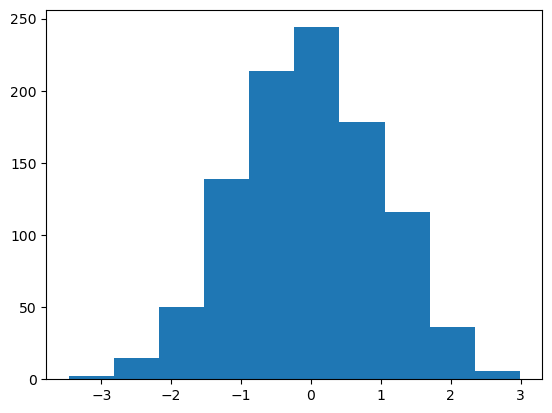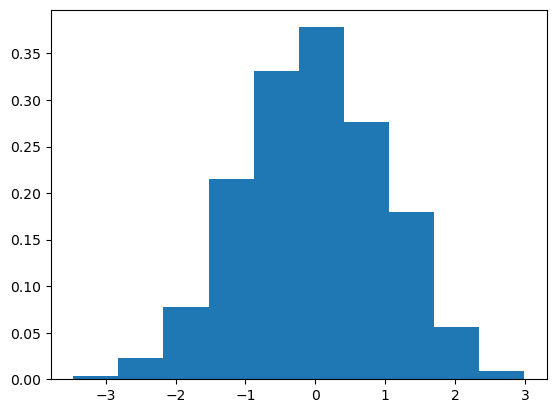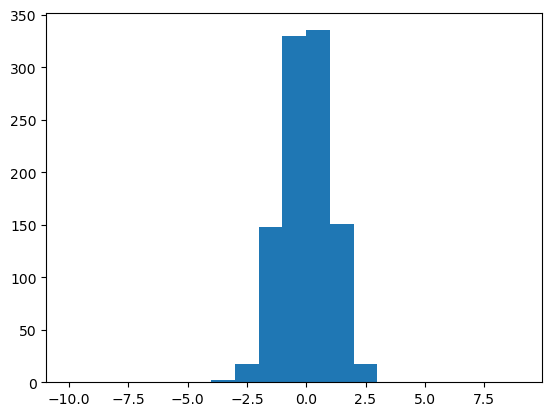# How to create a histogram (in Python, using Matplotlib)

See all solutions.

A histogram is a very common and useful data visualization. It displays an approximation of the distribution in single series of data points (one variable) by grouping the data into bins, each bin draw as a vertical bar. How can we create such a visualization?

Related topics:

## Solution

We will create some random data using NumPy, but that’s just for demonstration purposes. You can apply the answer below to any data, even if it’s stored just in plain Python lists.

1
2
import numpy as np
data = np.random.normal( size=1000 )


The conventional way to import matplotlib in Python is as follows.

1
import matplotlib.pyplot as plt


To create a histogram with 10 bins (the default):

1
2
plt.hist( data )  # or plt.hist( bins=20 ), or any number
plt.show()The $y$ axis in a histogram is frequency, or number of occurrences. You can change it to probabilities instead.

1
2
plt.hist( data, density=True )
plt.show()You can also choose your own bin boundaries.

1
2
plt.hist( data, bins=range(-10,10,1) )
plt.show()